# Effects of EGR and Alternative Fuels on Homogeneous Charge Compression Ignition (HCCI) Combustion Mode

Effects of EGR and Alternative Fuels on Homogeneous Charge Compression Ignition (HCCI) Combustion Mode
Laboratory of Mechanics and Energy (LME), Department of Mechanical Engineering, Faculty of Technology, University Hassiba Benbouali of Chlef, Chlef 02000, Algeria

Laboratory of Industrialist Fluid Measurement and Applications (FIMA), Department of Technology, Faculty of Science and Technology, University Djilali Bounaama of Khemis-Miliana, Khemis Miliana 44225, Algeria

Corresponding Author Email:
s.mohammedbelkebir@univ-chlef.dz
Page:
135-144
|
DOI:
https://doi.org/10.18280/ijdne.160203
Received:
19 November 2020
|
Accepted:
21 January 2021
|
Published:
30 April 2021
| Citation

OPEN ACCESS

Abstract:

We present in this article an analysis of the impacts of the exhaust gas recirculation (EGR) and alternative fuels on HCCI combustion mode. The objective is to reduce the pollutant emissions below the levels of established pollution standards. The ANSYS CHEMKIN-Pro software and the combined chemical kinetics mechanism were used to perform simulations for a closed homogeneous reactor under conditions relevant to HCCI engines. The calculation process is based on one single-zone in the combustion chamber. Numerical simulation has proven the ability of the models adopted, which use the essential mechanisms of the fuel combustion process, to reproduce, among other things, the evolution of the formation of chemical species. This study showed that adding hydrogen (H2) to methane (CH4) is an interesting alternative fuel because it reduces ignition time. It was concluded that an increase of EGR rate conducts to a slower combustion process, lower temperatures, and the reduction of nitrogen oxide (NOX) emissions.

Keywords:

pollutant emissions, direct injection diesel engine, HCCI engine, EGR, alternative fuel, chemical kinetics

1. Introduction

The diesel engine is considered among the most polluting sources and it is responsible for severe health human such as cancer and respiratory diseases . Successive models of diesel engines have been developed to reduce these emissions below pollution standards. Alternative fuels and EGR proportion related to the performance of diesel engine in a HCCI combustion mode are used in this study.

Conventional engines are characterized by heterogeneous combustion resulting in a propagation of a flame front and a high gas temperature responsible of NOX pollution. In these engines, the main pollutant emissions are reduced after combustion using external control systems . HCCI combustion mode is characterized by a direct action on mechanisms of pollutant formation. The combustion of the air-fuel mixture becomes highly homogeneous and instantaneous. One of the most techniques to reach this aim is to use technical exhaust gas recirculation (EGR) . The method is characterized by reintroducing burnt gas into the combustion chamber in order to lowering temperatures, delay auto-ignition after TDC, homogenize the mixture, and therefore the reduction of NOX rate and particulate emissions charaterized by a high heat release rate [4, 5].

The influence of several parameters on the HCCI combustion was investigated by Ghorbanpour and Rasekhi . The authors concluded that, the early injection generates a better homogeneous mixture and contribute to accelerate the transformation of fuel to lower hydrocarbons. Air-fuel ratio and the instant of ignition can control the reaction temperatures. Lower temperatures resulting from EGR delay the self-ignition and then can be very useful for specific diesel fuel with quick self-ignition leading to the early start combustion. Thus, the cooled EGR constitutes a fundamental tool in the start of combustion, lowering temperature and oxygen responsible for the appearance of NOX gas.

Supramani and Kumar  present several simulations using a review of differents processes to reach homogeneous charge in the combustion chamber. The CHEMKIN software was used to examine the impact of EGR on the operating parameters of HCCI engine combustion and on the emission pollutants [8, 9]. A decrease in UHC and CO emissions is due to the increasing of the intake temperature or compression ratio (CR). Furthermore, both reductions of the CR and the intake temperature retarded the auto-ignition timing .

The utilization of potential alternative fuels in an HCCI engine is to widen the operating regime [11, 12]. In Diesel engine, Ghazal  has presented a study on the use of methane in the fuel mixture for turbocharged combustion interne engines. It’s shown that methane added in the fuel has a significant effect on combustion and pollutant emission. Unfortunately, fraction methane increases pressure in the cylinder which leads to uncontrolled combustion. In addition, NO emission can be reduced significantly for 50% methane in the mixture.

Hydrogen is a potential alternative and promising in terms of high thermal efficiency and low emissions in HCCI combustion . Hora and Agarwal  then Kahraman et al.  show that adding hydrogen to natural gas (NG) increases the stability of combustion, contributes to enhance the efficiency of the engine and reduced emissions of CO2, CO and UHC while nitrogen monoxide (NO) increased with the hydrogen addition. Moreover, Pana et al.  have found that adding hydrogen causes an increase in NOX emissions, due to an increase in the gases temperature and the decrease in specific fuel consumption (SFC).

Alternative fuel in HCCI combustion mode is presented by Gowthaman and Sathiyagnanam . In this study combustion is controlled by optimum inlet air temperatures in order to show effect on NOX, CO and UHC emissions. The results show that NOX emission increases with the increase of the inlet temperature while the specific fuel consumption of HCCI engine decreased. Hasan et al.  have presented a numerical investigation of the HCCI engine modeled by a zero-dimensional single zone powered by a four stroke single cylinder diesel engine under the influence of several engine parameters. The means results show the importance of parameters such as intake air temperature and the engine speed on the HCCI combustion which is possible to be reached under very lean mixture.

The start of combustion (SOC) of a stoichiometric methane-air mixture in a single-zone combustion chamber is studied by Abareshi et al. . The results show the importance of initial temperature in controlling the SOC of HCCI engine. It’s concluded that SOC will not occur if the initial temperature is sufficiently low. Nishi et al.  present a study on the efficiency of HCCI engine under the effect of EGR ratio and engine speed. Results show that peak values of high temperature heat release reaction (HTHR) are decreased when EGR ratio increase.

Ennetta et al.  show the importance of adding hydrogen on the reduction in polluting emissions in HCCI single zone. The most important factor to make the combustion better homogeneous and instantaneous, the EGR is not considered in this study.

In the most common above cited references models are developed for partial analysis HCCI combustion. In this study a global model of HCCI combustion using simultaneously single zone model, specific for the homogeneous combustion, alternative fuel CH4/H2 and technical EGR is performed in order to reduce as much as possible the exhaust emissions in Diesel engine.

The present study is a real application of HCCI combustion under EGR effect with detailed chemical kinetics of a direct injection diesel F8L413 engine manufactured in Algeria.

2. Numerical Modeling

2.1 Model description

Figure 1 shows typical HCCI combustion where the fuel-air charge is fully premixed in order to make sufficiently homogeneous during inlet phase the HCCI engine involves a type of internal combustion in which the mixture of fuel takes place in the most homogenous way possible (as in gasoline engines), and is compressed strongly enough to reach the point of self-ignition where it ignites automatically. In phase combustion the mixture starts rapidly and simultaneously at multi points whenever heat and pressure are high enough (Figure 1b). In this study, this typical combustion HCCI is applied to direct injection diesel F8L413 engine in order to reduce emissions and protect the environment.

The HCCI combustion necessitates a grouping of three domain’s heat transfer, fluid mechanics, and chemical reaction. Single-zone HCCI combustion models allow detailed modeling of the chemical kinetics of practical fuels by assuming that the gas in the combustion chamber is homogeneous . Heywood  provides equations describing the volume as a function of engine parameters. The simulation model internal combustion engine (ICE), which is a closed system 0-D, is valid in the period where intake and exhaust valves are closed. The calculation process on a single-zone is simulated in CHEMKIN software in this work. The input parameters used are: the start crank angle equal to -180 deg before the top dead center (TDC); the cycle end time equal to 180 deg after TDC; the engine speed equal to 2600 rpm; the gas mixture pressure at intake valve close (IVC) equal to 1.2 bar; the gas mixture temperature at IVC equal to 460 K; the equivalence ratio (ɸ) equal to 0.3. In addition, the heat transfer correlation has as parameters: a=0.035, m=0.7, c=0.0; the temperature of the wall is taken equal to 400 K; the Woschni correlation of average cylinder gas velocity has as parameters: C11=2.28, C12= 0.308, C2=3.24x10-3 and finally the swirl speed ratio is taken equal to 0.0. The amount of gas due to the EGR introduced into the simulation by CHEMKIN and consists of CO2, H2O, N2 and O2. Its quantity varied from 0% to 60% on the one hand and on the other hand, the quantity of H2 added to CH4 varied from 0% to 40%.

## 1a.png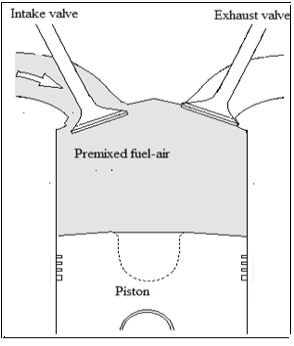(a) Premixed fuel

## 1b.png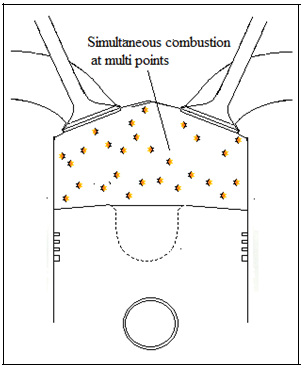(b) Homogeneous charge compression ignition

Figure 1. Typical HCCI with premixed fuel air for F8L413 engine

The characteristics of the F8L413 engine and the operating conditions are given in Table 1.

Table 1. Simulated engine specifications

 Characteristics Specification Type of engine Nominal max speed Bore (mm) Stroke (mm) Displacement (cm3) Compression ratio Connecting rod length (mm) Fuel type compression ignition 2650 rpm 120 125 11310 18: 1 238 n-hexadecane (C16H34)

The single-zone HCCI thermodynamic simulation model is used.

The following assumptions are made:

• Temperature and pressure have a uniform distribution;
• The heat exchange is related to the walls and is taken into account by the modified Woschni model ;
• The fuel and air are premixed outside of the cylinder;
• The mixture ignites automatically by auto-ignition;
• Mixing in the cylinder is considered as ideal gas.

2.2 Numerical tool

CHEMKIN is a set of software tools, written in Fortran language, to solve complex problems in chemical kinetics in the gas phase. Thermochemical data for each species in the chemical kinetic mechanism is used to calculate thermodynamic properties, heat transfer properties, and reaction equilibrium constants .

2.3 Chemical kinetics model

The CHEMKIN-Pro software is designed for modeling different chemically reacting flow configurations . Therefore, CHEMKIN-Pro was used as the calculation solver.

The "I" reversible (or irreversible) surface reactions involve "K" chemical species can be represented in the general form :

$\sum_{k=1}^{K} v_{k i}^{\prime} X_{k} \Leftrightarrow \sum_{k=1}^{K} v_{k i}^{\prime \prime} X_{k} \quad(i=1, \ldots, I)$  (1)

$\dot{\omega}_{k}=\sum_{i=1}^{I}\left(v_{k i}^{\prime \prime}-v_{k i}^{\prime}\right) q_{i} \quad(k=1, \ldots \ldots \ldots, K)$  (2)

$q_{i}=k_{f i} \Pi_{k=1}^{K}\left[X_{k}\right]^{v_{ki}^{\prime}}-k_{r i} \Pi_{k=1}^{K}\left[X_{k}\right]^{v_{ki}^{\prime\prime}}$  (3)

where:

K: Number of chemical species, dimensionless;

[Xk]: Molar concentration of the kth species, mole/cm3;

$\dot{\omega}_{k}$: The chemical production rate of the kth species due to gas-phase reactions, mole/cm3/sec;

qi: Rate-of-progress variable, mole/cm3/sec;

$v_{k i}^{\prime}$ and $v_{k i}^{\prime \prime}$ represent the stoichiometric coefficients of the kth reactant species and product species in the ith reaction, respectively.

The forward "kfi" and reverse rate "kri" constants and for the "I" reactions are expressed by:

$k_{f i}=A_{i} T^{\beta i} \exp \left(\frac{-E_{i}}{R_{c} T}\right)$  (4)

$k_{r i}=\frac{k_{f i}}{K_{c i}}$  (5)

where:

Ai: Pre-exponential factor;

βi: Temperature exponent;

Ei: Activation energy for the reaction;

Rc: Universal gas constant;

Kci: Equilibrium constant in concentration units for the ith reaction.

2.4 Governing equations

The governing equations for temperature and species are given as follows:

• Internal Energy/Temperature:

$\rho C_{v} \frac{d T}{d t}=-\sum_{k=1}^{k g a s} \dot{\omega}_{k} W_{k} u_{k}-\frac{P}{V} \frac{d V}{d t}-\frac{h_{g}\left(T-T_{w}\right) A_{w}}{V}$  (6)

-   Species conservation equation with constant mass:

$\rho \frac{d Y_{k}}{d t}=\dot{\omega}_{k} W_{k}$  (7)

with:

P: Pressure cylinder, bar;

Tw: Chamber wall temperature, K;

V: Volume, m3;

Yk: Mass fraction of the kth species, dimensionless;

Cv: Constant-volume specific heat capacity of the mixture, J/kg/ K;

T: Temperature, K;

ρ: Mass density of a gas mixture, kg/m3;

uk: Specific internal energy of the kth species, J/kg;

Wk: Molecular weight of the kth species, kg/mole;

hg: Heat transfer coefficient, W/m2/K;

Aw: Surface area, m2.

2.5 Test fuels used for HCCI combustion

The complete stoichiometric combustion reaction for a lean mixture is written as:

$C_{x} H_{y}+\frac{a_{s t}}{\varphi}\left(O_{2}+3.76 N_{2}\right)$

$\rightarrow x C O_{2}+\frac{y}{2} H_{2} O+\gamma O_{2}+3.76 \frac{a_{s t}}{\varphi} N_{2}$  (8)

with:

$a_{s t}=x+\frac{y}{4}$

ɸ: Equivalence ratio, dimensionless.

(1) Natural gas (methane) and gas mixture

Methane fueled HCCI engines operate at high CR  (15: 1 to 21: 1), resulting in high efficiency. Methane is considered a representative of natural gas; its chemical kinetic mechanism is incorporated into the GRI-MECH-3.0 mechanism , which takes into account 53 species and 325 reactions, including the chemistry of NOX. As part of this study, we used the internal combustion engine (ICE) model to follow the performance parameters as a versus of the crank angle and the different hydrogen fractions (10%, 20%, 30% and 40%) in methane-hydrogen mixtures at constant equivalence ratio (ɸ) equal to 0.3 and engine speed equal to 2600rpm.

Mixture (CH4+H2) combustion reaction is written as follows,

$(1-\alpha) C H_{4}+\alpha H_{2}+$

$\left(\frac{2}{\phi}(1-\alpha)+\frac{\alpha}{2 \phi}\right)\left(O_{2}+3.762 N_{2}\right)$   (9)

where the molar fraction of hydrogen added "α" is given by:

$\alpha=\frac{n\left(H_{2}\right)}{n\left(H_{2}\right)+n\left(C H_{4}\right)}$  (10)

where, n(H2) and n(CH4) are respectively the number of moles of hydrogen and methane, knowing that total molar fraction of fuel (methane+hydrogen) is the unit.

(2) N-heptane fuel

N-heptane (n-C7H16) is a fuel usable for HCCI engines [31, 32], it was chosen for cetane number (CN~56) which is quite close that of conventional diesel fuels (CN~50) .

Table 2 shows some compared properties of different fuels.

Table 2. Comparative properties of hydrogen, methane, n-hexadecane and n-heptane

 Properties H2 CH4 C16H34 C7H16 Molar mass (kg/kmol) 2.02 16.04 226.43 100.21 LHV (MJ/kg) 119.9 50 44.07 44.92 Octane number >130 >120 - - Cetane number - - 100 56
3. Results and Discussion

The "CHEMKIN-Pro" package is used to model the combustion and the chemical kinetics of HCCI engine. This software is one of the most popular software packages for the history of the net heat release rate (NHRR), temperature, pressure of the cylinder and species concentrations. This paper provides a study of the effect of different fuels and EGR rate on the HCCI combustion engine and emissions. The simulation results for different fuels such as n-heptane, methane, hydrogen and the mixture (methane+hydrogen) were compared with n-hexadecane (C16H34) base fuel which fueling the F8L413 engine. The analysis results are interesting in terms of performance and emissions. We discuss below the different emissions and performance criteria for the fuel studied.

The analysis of Figure 2 shows that the addition of hydrogen leads to an increase in the ignition advance of the mixture. As the proportion of hydrogen in the mixture is increased, the moment of self-ignition is advanced. The explanation is that for high hydrogen fractions are added to the mixture, the reaction becomes explosive on the one hand, and on the other hand, pressure (see Figure 3a) and temperatures (see Figure 2) significant levels can be reached . In addition, when the hydrogen does not contain carbon (C), the reactive chain of its combustion is shorter which causes an advanced self-ignition moment and a shorter ignition time .

## 2.jpg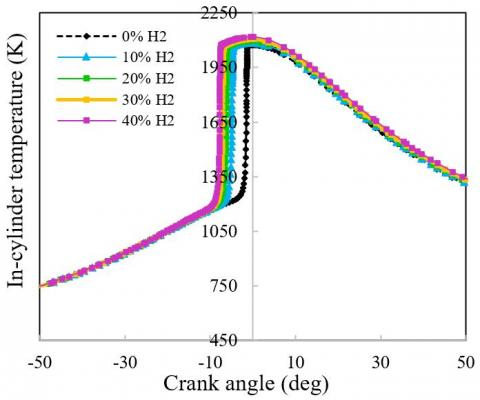Figure 2. Temperature variation for different molar fractions of hydrogen

## 3a.jpg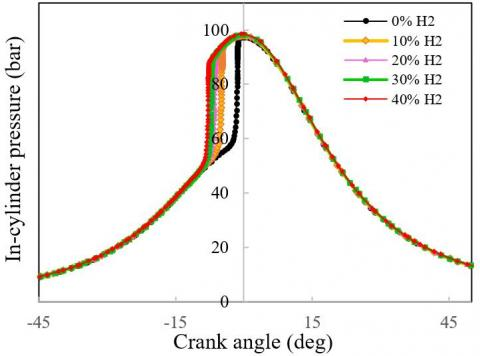(a) Pressure variation for different molar fractions of H2 at ɸ = 0.3

## 3b.jpg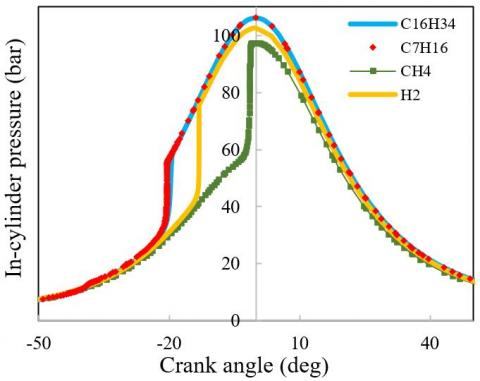(b) Pressure variation for different fuels

Figure 3. Pressure evolution for different fuels

## 4a.jpg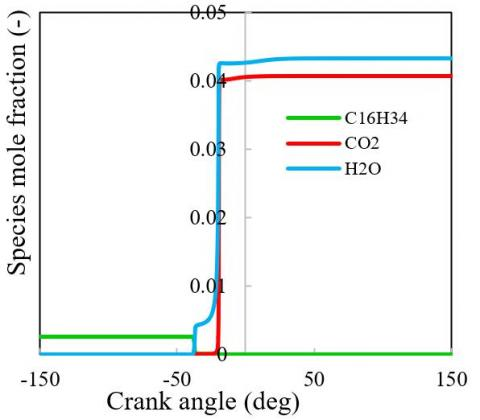(a) 100% C16H34

## 4b.jpg(b) 100% CH4

## 4c.jpg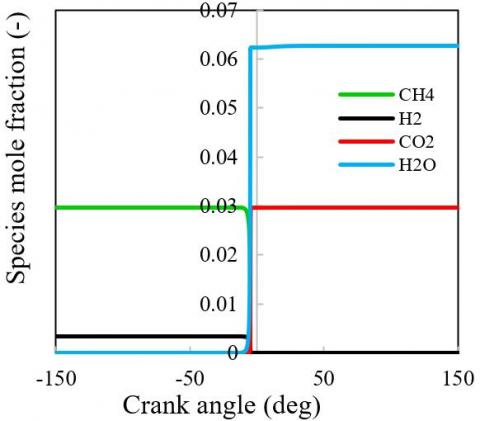(c) 90% CH4

## 4d.jpg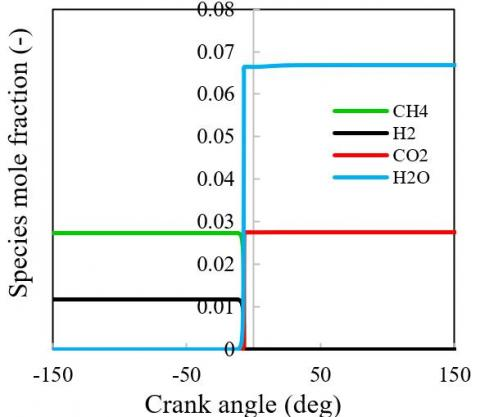(d) 70% CH4

## 4e.jpg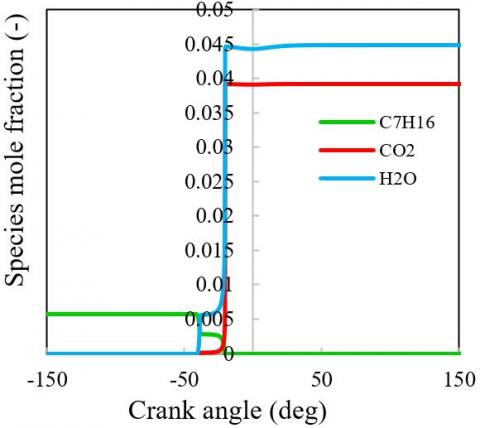(e) 100% C7H16

## 4f.jpg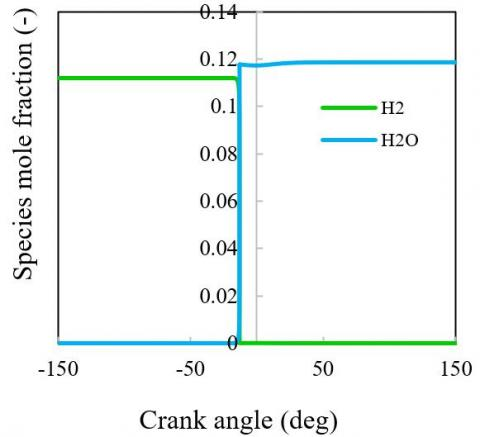(f) 100% H2

Figure 4. Species mole fraction evolution for different fuels

The pressure variation in the cylinder for different fuels types used is illustrated in Figure 3b. It was noted that the maximum gas pressure in the combustion chamber of F8L413 engine for n-hexadecane fuel is high compared to the other fuels used. This is due to the highest cetane number of n-hexadecane, i.e. 100.

Figure 4 shows the molar concentrations of the production and disappearance of the majority species during combustion operation for different fuels under lean stoichiometric conditions. It was noted that an increase in water production (H2O) with hydrogen enrichment. As the mixture is compressed, the gas temperature in the combustion chamber gradually increases. The species concentration begins to vary and then CO and NO begin to form gradually. The CO2 is an inevitable gas present in the combustion products and according to the simulation results obtained, the CO2 is indeed produced. However, replacing a fraction of methane with hydrogen leads to a reduction in the production of CO (see Figure 5a) and CO2 (see Figure 4d)  on the one hand, and on the other hand an increase occurs in the NHRR (see Figure 5b). This can be explained by the fact that, adding hydrogen to the mixture increases the stability of combustion

The EGR are mainly composed of CO2, H2O, O2 and N2. Therefore, it is believed to have an effect on the combustion process. Figure 6a shows the variation of NHRR with respect to crank angle for different EGR rates. It was noted that from this Figure, the total duration of combustion increased significantly with the EGR rate. The peak of the NHRR per crank angle decreases rapidly when the EGR rate goes from 0% to 60%. Once a rate of CO2 leaves the engine with the exhaust gases, it is not very reactive or flammable and can therefore be used as an additive to slow combustion. Moreover, these results are related to the in-flammable water.

## 5a.jpg(a)

## 5b.jpg(b)

Figure 5. Variation of (a) CO molar fraction; and (b) net heat release rate for different molar fractions of hydrogen

## 6a.jpg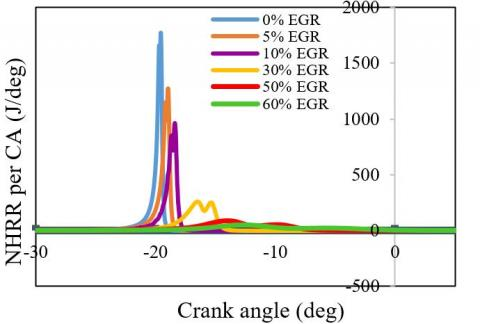(a)

## 6b.jpg(b)

## 6c.jpg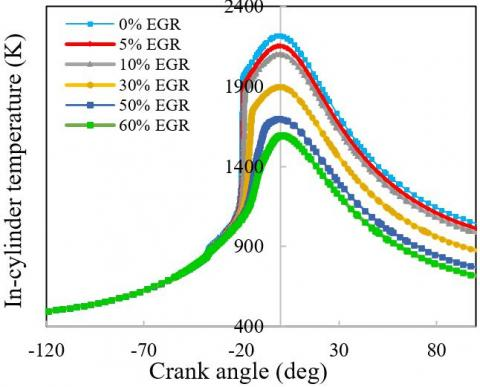(c)

Figure 6. Evolution of (a) NHRR; (b) pressure and; (c) temperature cylinder for C16H34 fuel for different EGR rates

## 7a.jpg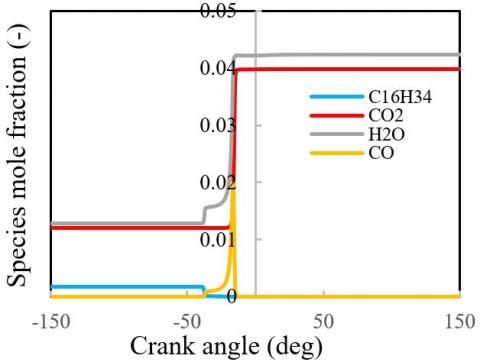(a) 5% EGR

## 7b.jpg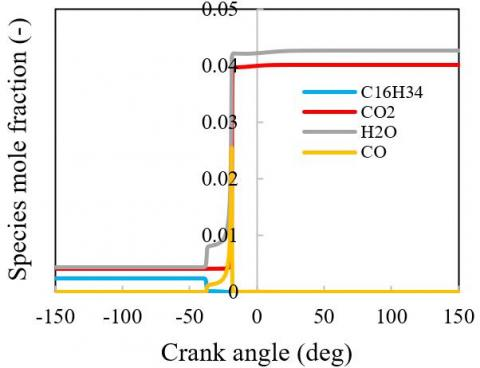(b) 10% EGR

## 7c.jpg(c) 30% EGR

## 7d.jpg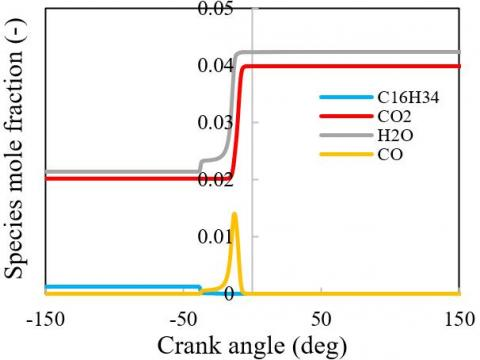(d) 50% EGR

## 7e.jpg(e) 60% EGR

Figure 7. Species molar concentrations evolution for different EGR rates

## 8.jpg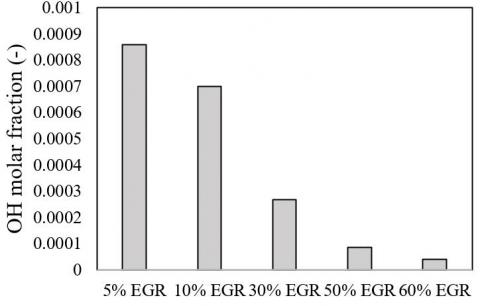Figure 8. OH molar fraction variation for different EGR rates

## 9a.jpg(a) NO molar fraction evolution for different molar fractions of hydrogen at ɸ = 0.3

## 9b.jpg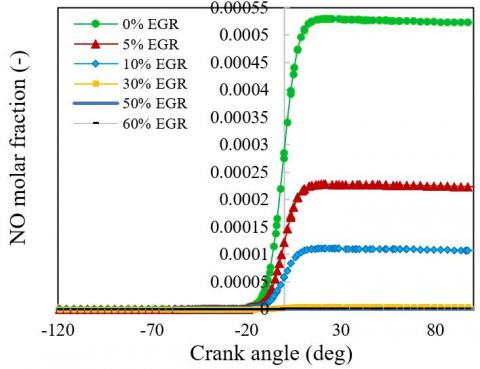(b) NO molar fraction evolution for different EGR rates

Figure 9. NO molar fraction evolution

It has been shown that water vapor can be added to HCCI combustion to slow the combustion rate and thus increase the high load limits . The heat rate generation from the fuel generates a variation in the pressure and temperature of the gas in the engine cylinder. Therefore, the increase in the rate of EGR causes a significant decrease in temperature (see Figure 6c), pressure (see Figure 6b) and carbon monoxide production (see Figure 7). The explanation for this can be due to the higher thermal capacity of the exhaust gases, which delays the mixture to the required conditions for the initiation the cool flame combustion .

Figure 9 represents the variation of NO. Figure 9a shows that the addition of hydrogen causes an increase in NOX emissions due to an increase in the combustion temperature and a decrease in the SFC . While UHC (see Figure 10a) and CO (see Figure 5a) emissions were reduced . Figure 9b shows the NO molar fraction versus crank angle with different EGR rates. It can be seen that with the increase in the rate of EGR, NO emissions are clearly reduced compared to those of combustion without EGR. In addition, the increase in the EGR rate leads to a reduction in the OH molar fraction (see Figure 8). The reason for this can be attributed to the fact that the increase of the recycled gases, reduces NHRR, and thus lowers the maximum temperature of the cylinder due mainly to CO2 and H2O, which have higher specific calorific capacities . The decrease in temperature and pressure cylinder with the increase in the EGR rate leads to the reduction of the indicated specific fuel consumption (ISFC), torque, indicated work, and therefore an increase in the indicated thermal efficiency as shown in Table 4.

Figure 10a represents the evolution of the UHC molar fraction. It can be seen that the UHC emissions decrease with the increase of hydrogen fraction and decreased significantly with the increase of EGR rate as shown in Figure 10b.

The maximum torque generated by methane is greater than that generated by the other fuels tested. It reaches its maximum value at 52.91(N. m) as shown in Table 3. Thus, the indicated thermal efficiency of the mixture at 40% hydrogen is 6.56% higher compared to a mixture 0% hydrogen (pure methane) and 18.32% compared to diesel fuel (n-hexadecane). In addition, the ISFC is reduced by 23.04% and 28.10% respectively for a mixture with 0% of hydrogen and a mixture with 40% of hydrogen compared to n-hexadecane fuel. This can be explained by the fact that hydrogen has more energy than methane and its lower heating value (LHV=120 MJ/kg) is higher than other types of fuel used .

The numerical simulation results obtained clearly show that the type of fuel has a significant effect on the temperature and pressure gases in the combustion chamber, therefore on emissions as well as on engine performance as indicated in Tables 3 and 4.

## 10a.jpg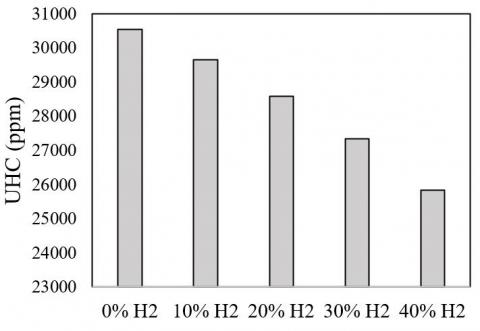(a) UHC for different molar fractions of H2 at ɸ = 0.3

## 10b.jpg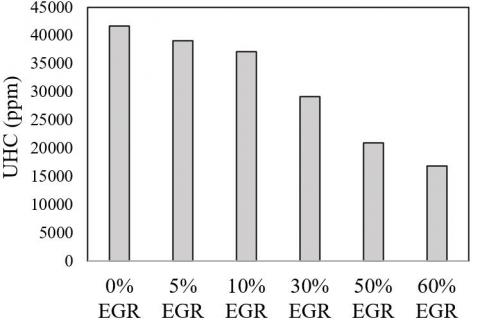(b) UHC evolution with different EGR rates

Figure 10. UHC evolution

Table 3. Engine performance parameters (cycle) with different fuels

 Fuel Indicated work (kJ) IMEP (bar) Indicated power (kW) Torque (N. m) ISFC (g/KW-h) Indicated thermal efficiency (-) C16H34 0.6146 4.348 13.32 48.91 161.9 0.5052 C7H16 0.5918 4.186 12.82 47.09 163.2 0.4911 CH4 0.6649 4.703 14.41 52.91 124.6 0.5779 H2 0.6533 4.621 14.16 51.99 58.38 0.5139 10%H2 -90%CH4 0.6581 4.655 14.26 52.37 123.9 0.5813 20%H2- 80%CH4 0.6547 4.631 14.19 52.10 122.2 0.5894 30%H2- 70%CH4 0.6530 4.619 14.15 51.97 119.7 0.6016 40%H2- 60%CH4 0.6524 4.615 14.14 51.92 116.4 0.6185

Table 4. Engine performance parameters (cycle) with different EGR rates

 Performance 0 %-EGR 5 %-EGR 10 %-EGR 30 %-EGR 50 %-EGR 60 %-EGR Max-T (K) 2216.0 2154.0 2103.0 1899.0 1698.0 1595.0 Max-P (bar) 106.1 103.0 100.5 90.36 80.49 75.46 Indicated work (kJ) 0.6146 0.5816 0.5537 0.4395 0.3239 0.263 Torque (N.m) 48.91 46.28 44.06 34.97 25.78 20.93 ISFC (g/kWh) 161.9 160.4 159.7 156.4 151.6 149.4 Indicated thermal efficiency (-) 0.5052 0.5101 0.5124 0.5232 0.5397 0.5478
4. Conclusions

ANSYS CHEMKIN-Pro software is widely used in the development and optimization of combustion. Several tests of the calculation code were done. The general conclusions can be summarized as follows:

1. The addition of H2 to CH4 has a significant effect on the HCCI combustion engine mode.

2. The effect of adding H2 to CH4 results a reduction in fuel consumption and burning time on the one hand and on the other hand, the indicated thermal efficiency was increased.

3. Improving the engine thermal efficiency and reducing CO and UHC emissions can be achieved by adding a certain percentage of H2 to the lean methane. Hydrogen therefore represents an element of self-ignition control of (CH4+H2) mixtures.

4. When the initial conditions are known, the single-zone model makes it possible to accurately predict the ignition of an HCCI engine.

5. Pollutants formation such as CO and NO in the combustion chamber, among other things, is associated with the presence of high temperatures in the flame.

6. Increasing the EGR rate slows the combustion process, resulting in a lower rate of heat release rate and thus a longer burning rate. In addition, it contributes to reducing NO emissions. The NOX was reduced by 52.42% for (10%-EGR) compared to (5%-EGR) used.

Results have shown that among the considered parameters, the mixture (methane+hydrogen) is one of the best alternatives as a renewable fuel for diesel engines from economic point of view, energy and environmental protection while overcoming difficulties in infrastructures, development, production and distribution of hydrogen.

Acknowledgment

The authors would like to thank all who helped enrich this work, either directly or indirectly, and in particular, members of the Mechanical Energy Laboratory at Chlef University and the DGRSDT, Algiers, contribution.

Nomenclature
 CA Crank Angle deg Degrees IMEP Indicated Mean Effective Pressure UHC Unburned Hydrocarbons CO Carbon Monoxide CO2 Carbon Dioxide rpm Revolution Per Minute
References

 Steiner, S., Bisig, C., Petri-Fink, A., Rothen-Rutishauser, B. (2016). Diesel exhaust: current knowledge of adverse effects and underlying cellular mechanisms. Archives of Toxicology, 90(7): 1541-1553. https://doi.org/10.1007/s00204-016-1736-5

 Reşitoğlu, İ.A., Altinişik, K., Keskin, A. (2015). The pollutant emissions from diesel-engine vehicles and exhaust aftertreatment systems. Clean Technologies and Environmental Policy, 17(1): 15-27. https://doi.org/10.1007/s10098-014-0793-9.

 Ladommatos, N., Adelhalim, S.M., Zhao, H., Hu, Z. (1998). The effects of carbon dioxide in exhaust gas recirculation on diesel engine emissions. Proceedings of the Institution of Mechanical Engineers, Part D: Journal of Automobile Engineering, 212(1): 25-42. https://doi.org/10.1243/0954407981525777

 Jacobs, T., Assanis, D., Filipi, Z. (2003). The impact of exhaust gas recirculation on performance and emissions of a heavy-duty diesel engine. SAE Technical paper. https://doi.org/10.4271/2003-01-1068

 Hampson, G.J. (2005). Heat release design method for HCCI in diesel engines. SAE Technical Paper. https://doi.org/10.4271/2005-01-3728.

 Ghorbanpour, M., Rasekhi, R. (2013). A parametric investigation of HCCI combustion to reduce emissions and improve efficiency using a CFD model approach. Fuel, 106: 157-165. https://doi.org/10.1016/j.fuel.2012.12.008

 Supramani, S., Kumar, C. (2019). Impacts on Nox emission control measures to achieve EURO VI limits - A Review. Journal Européen des Systèmes Automatisés, 52(2): 16-171. https://doi.org/10.18280/jesa.520208

 Benhorma, S., Aouissi, M., Mansour, C., Bounif, A. (2017). Contribution to study the effect of exhaust gas recirculation EGR on HCCI combustion mode. International Journal of Heat and Technology, 35(1): 183-190. https://doi.org/10.18280/ijht.350124

 Jamsran, N., Lim, O. (2016). Effects of EGR and boosting on the auto-ignition characteristics of HCCI combustion fueled with natural gas. Journal of Natural Gas Science and Engineering, 35: 1015-1024. https://doi.org/10.1016/j.jngse.2016.09.016

 Peng, Z., Zhao, H., Ma, T., Ladommatos, N. (2005). Characteristics of homogeneous charge compression ignition (HCCI) combustion and emissions of n-heptane. Combustion Science and Technology, 177(11): 2113-2150. https://doi.org/10.1080/00102200500240588

 Iida, N. (1997). Alternative fuels and homogeneous charge compression ignition combustion technology. SAE Technical Paper, 978449.

 Hasan, M.M., Rahman, M.M., Kadirgama, K. (2015). A review on homogeneous charge compression ignition engine performance using biodiesel-diesel blend as a fuel. International Journal of Automotive and Mechanical Engineering, 11: 2199. http://dx.doi.org/10.15282/ijame.11.2015.3.0184

 Ghazal, O. (2018). Air pollution reduction and environment protection using methane fuel for turbocharged ci engines. Journal of Ecological Engineering, 19(5): 52-58. https://doi.org/10.12911/22998993/91276

 brahim, M.M., Ramesh, A. (2014). Investigations on the effects of intake temperature and charge dilution in a hydrogen fueled HCCI engine. International Journal of Hydrogen Energy, 39(26): 14097-14108. https://doi.org/10.1016/j.ijhydene.2014.07.019

 Hora, T., Agarwal, A. (2015). Experimental study of the composition of hydrogen enriched compressed natural gas on engine performance, combustion and emission characteristics. Fuel, 160: 470-478. https://doi.org/10.1016/j.fuel.2015.07.078

 Kahraman, N., Ceper, B., Akansu, S., Aydin, K. (2009). Investigation of combustion characteristics and emissions in a spark-ignition engine fuelled with natural gas-hydrogen blends. International Journal of Hydrogen Energy, 34(2): 1026-1034. https://doi.org/10.1016/j.ijhydene.2008.10.075

 Pana, C., Negurescu, N., Popa, M., Cernat, A., Soare, D. (2007). An investigation of the hydrogen addition effects to gasoline fueled spark ignition engine. SAE Technical Paper. https://doi.org/10.4271/2007-01-1468

 Gowthaman, S., Sathiyagnanam, A. (2018). Analysis the optimum inlet air temperature for controlling homogeneous charge compression ignition (HCCI) engine. Alexandria Engineering Journal, 57(4): 2209-2214. https://doi.org/10.1016/j.aej.2017.08.011

 Hasan, M., Rahman, M., Kadirgama, K., Ramasamy, D. (2018). Numerical study of engine parameters on combustion and performance characteristics in an n-heptane fueled HCCI engine. Applied Thermal Engineering, 128: 1464-1475. https://doi.org/10.1016/j.applthermaleng.2017.09.121

 Abareshi, B., Mohagheghi, H., Kalalabadi, M. (2017). Investigate auto-ignition of stoichiometric methane-air mixture. 5th International Fule and Combustion Conference December 8.

 Nishi, M., Kanehara, M., Iida, N. (2016). Assessment for innovative combustion on HCCI engine by controlling EGR ratio and engine speed. Applied Thermal Engineering, 99: 42-60. https://doi.org/10.1016/j.applthermaleng.2015.11.126

 Ennetta, R., Yahya, A., Said, R. (2012). Etude de la combustion du méthane enrichi à l’hydrogène. 2ème Conférence Maghrébine sur les Matériaux et l’Energie à Tozeur.

 Chemkin, A. (2015). Ansys chemkin theory manual 17.0 (15151). San diego: reaction design.

 Heywood, J. (1988). Internal Combustion Engine Fundamentals. McGraw-Hill, Inc.

 Chang, J., Guralp, O., Filipi, Z., Assanis, D., Kuo, T., Najt, P., Rask, R. (2004). New heat transfer correlation for an HCCI engine derived from measurements of instantaneous surface heat flux. SAE Transactions, 113: 1576-1593. https://www.jstor.org/stable/44723615

 Seddak, M., Liazid, A. (2016). The effects of using a biofuel on the performance of a marine diesel engine. Mathematical Modelling of Engineering Problems, 3(4): 195-197. https://doi.org/10.18280/mmep.030408

 Design, R. (2011). CHEMKIN Tutorials Manual CHEMKIN® Software. (10112/15112), 1-274.

 Coltrin, M., Kee, R., Rupley, F. (1991). Surface chemkin (version 4.0): A fortran package for analyzing heterogeneous chemical kinetics at a solid-surface—gas-phase interface. Sandia National Labs., Livermore, CA (United States). https://doi.org/10.2172/6128661

 Olsson, J., Tunestål, P., Johansson, B., Fiveland, S., Agama, R., Willi, M., Assanis, D. (2002). Compression ratio influence on maximum load of a natural gas fueled HCCI engine. SAE Transactions, 111: 442-458. https://www.jstor.org/stable/44743073

 Smith, G.P. (1999). GRI-Mech 3.0. http://www.me.berkeley.edu/gri_mech, accessed on 1 June 2011.

 Zheng, Z., Yao, M. (2006). Numerical study on the chemical reaction kinetics of n-heptane for HCCI combustion process. Fuel, 85(17-18): 2605-2615. https://doi.org/10.1016/j.fuel.2006.05.005

 Peng, Z., Zhao, H., Ladommatos, N. (2003). Effects of air/fuel ratios and EGR rates on HCCI combustion of n-heptane, a diesel type fuel. SAE Technical Paper. https://doi.org/10.4271/2003-01-0747

 Boumeddane, B. (2009). Investigations numériques de l'auto inflammation des mélanges méthane/air en mode HCCI. Congrès français de mécanique. AFM, Maison de la Mécanique, 39/41 rue Louis Blanc-92400 Courbevoie. http://hdl.handle.net/2042/36700

 Ouksel, T., Chelghoum, A., Mameri, A. (2017). Etude de la combustion HCCI du GNC enrichi à l’hydrogène à l’aide de chemkin-Pro. Revue des Energies Renouvelables, 20(3): 423-431.

 Correia, E. (2010). Computational studies on HCCI engines. MPhil Thesis, Cranfield University. http://dspace.lib.cranfield.ac.uk/handle/1826/7782

 Ouksel, T., Chelghoum, A., Mameri, A. (2016). Numerical investigation of the effect of cooled EGR on turbocharger HCCI engine performance fueled with methane. International Journal of Automotive Engineering, 7(1). http://dx.doi.org/10.22068/ijae.7.1.2300